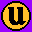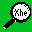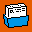# Setting access permissions numerically

There is a shorthand way of setting permissions by using octal numbers. Read permission is given the value 4, write permission the value 2 and execute permission 1.

```    r  w  x
4  2  1
```

These values are added together for any one user category:

```    1   =   execute only
2   =   write only
3   =   write and execute (1+2)
5   =   read and execute (4+1)
6   =   read and write (4+2)
7   =   read and write and execute (4+2+1)
```

So access permissions can be expressed as three digits. For example:

```                          user    group   others

chmod 640 file1       rw-     r--     ---
chmod 754 file1       rwx     r-x     r--
chmod 664 file1       rw-     rw-     r--
```